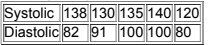×
Get Full Access to Elementary Statistics - 12 Edition - Chapter 10.6 - Problem 10 cqq
Get Full Access to Elementary Statistics - 12 Edition - Chapter 10.6 - Problem 10 cqq

×

# The exercises are based on the following sample dataISBN: 9780321836960 18

## Solution for problem 10 CQQ Chapter 10.6

Elementary Statistics | 12th Edition

• Textbook Solutions
• 2901 Step-by-step solutions solved by professors and subject experts
• Get 24/7 help from StudySoup virtual teaching assistantsElementary Statistics | 12th Edition

4 5 1 322 Reviews
21
1
Problem 10 CQQ

The exercises are based on the following sample data obtained from different second­year medical students who took blood pressure measurements of the same person (based on data from Marc Triola, MD).If the sample data were to result in the scatterplot shown here, what is the value of the linear correlation coefficient r?Step-by-Step Solution:

Step 1 of 1If the sample data were to result in the scatterplot shown here,the value of the linear correlation coefficient r = -1.

Step 2 of 1

##### ISBN: 9780321836960

This full solution covers the following key subjects: data, sample, based, medical, cqq. This expansive textbook survival guide covers 121 chapters, and 3629 solutions. The full step-by-step solution to problem: 10 CQQ from chapter: 10.6 was answered by , our top Statistics solution expert on 03/15/17, 10:30PM. Since the solution to 10 CQQ from 10.6 chapter was answered, more than 353 students have viewed the full step-by-step answer. Elementary Statistics was written by and is associated to the ISBN: 9780321836960. This textbook survival guide was created for the textbook: Elementary Statistics, edition: 12. The answer to “?The exercises are based on the following sample data obtained from different second­year medical students who took blood pressure measurements of the same person (based on data from Marc Triola, MD). If the sample data were to result in the scatterplot shown here, what is the value of the linear correlation coefficient r?” is broken down into a number of easy to follow steps, and 53 words.

Unlock Textbook Solution